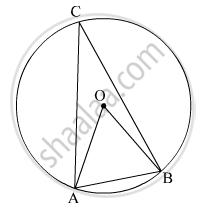Advertisement Remove all ads

# In the given figure, in a circle with centre O, length of chord AB is equal to the radius of the circle. Find measure of each of the following. (1) ∠ AOB (2)∠ ACB (3) arc AB (4) arc ACB. - Geometry

Sum

In the given figure, in a circle with centre O, length of chord AB is equal to the radius of the circle. Find measure of each of the following.
(1) ∠ AOB (2)∠ ACB
(3) arc AB (4) arc ACB.Advertisement Remove all ads

#### Solution

(1) In the given figure, OA and OB are the radii of the circle.
OA = OB = AB        (Given)
∴ ∆OAB is an equilateral triangle.
⇒ ∠AOB = ∠OAB = ∠OBA = 60º
Thus, the measure of ∠AOB is 60º.
(2) The measure of an angle subtended by an arc at a point on the circle is half of the measure of the angle subtended by the arc at the centre.
∠ACB = $\frac{1}{2}$ ∠AOB = $\frac{1}{2}$ x 60° = 30°
Thus, the measure of ∠ACB is 30º.
(3) m(arc AB) = ∠AOB = 60º       (Measure of an arc is the measure of its corresponding central angle)
Thus, the measure of arc AB is 60º.
(4) m(arc ACB) = 360º − m(arc AB) = 360º − 60º = 300º
Thus, the measure of arc ACB is 300º.

Concept: Property of Sum of Measures of Arcs
Is there an error in this question or solution?
Advertisement Remove all ads

#### APPEARS IN

Advertisement Remove all ads
Advertisement Remove all ads
Share
Notifications

View all notifications

Forgot password?